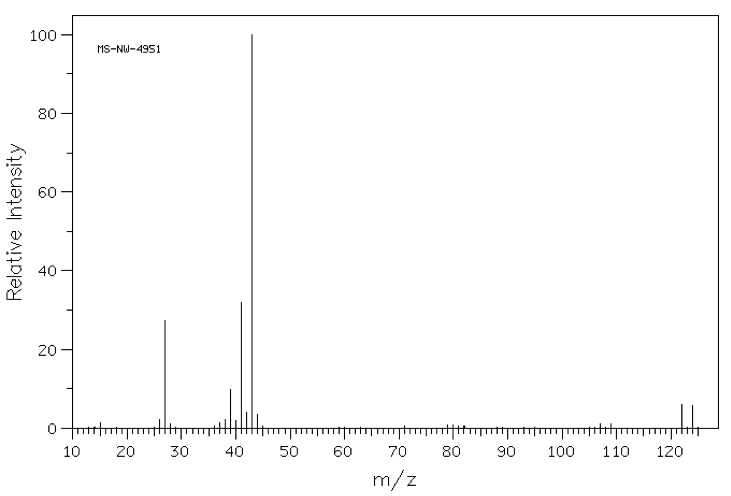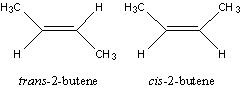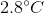Organic Chemistry : How to Quantify Compounds

Example Questions

Example Question #41 : Organic Chemistry

A chemist places a sample into a mass spectrometer and obtains the following spectrum that features a parent ion of 122 m/z as shown below. What element, in addition to carbon and hydrogen, must be present in her sample?Chlorine

Sulfur

Bromine

Oxygen

Phosphorus

Bromine

Explanation:

The basic principle being tested here is that mass spectra show the exact mass of each molecular fragment (originally derived from the sample) detected in the instrument. This means that, while we often think of the atomic mass of elements as a weighted average of the masses of the respective isotopes of an element, mass spectrometry reveals the isotopic variances in these fragments.

The correct answer is bromine, as indicated by the "doublet" feature centered at 121 m/z units. This doublet arises due to the fact that 79Br and 81Br each occur naturally in equal abundance; thus half of the molecules in a monobrominated sample will contain a 79Br atom, giving rise to the peak at the lower m/z value, and the other half, containing an 81Br, will give rise to a peak two m/z units higher.

Another important elemental mass spectrum signature to remember is that of chlorine, which occurs as 35Cl (75% abundance) and 37Cl( 25% abundance). A mass spectrum of a molecule containing chlorine will give a similar doublet, but the lower m/z value peak will be three times as large as the peak two mass units higher.

Example Question #1 : How To Quantify Compounds

A reaction is performed that produces a mixture of cis- and trans-2-butene. What is the best analytical method to quantify the ratio of these isomers (shown)?H NMR

Mass spectrometry

Infrared spectroscopy

Thin layer chromatography

Gas chromatography

The two alkenes have a boiling point difference ofand are easily separated by gas chromatography. The area under the curve on the chromatogram is proportional to the amount of each isomer.  H NMR is an excellent method to qualitatively analyze the isomers, but is not sensitive enough to quantitatively analyze the isomers compared to gas chromatography. Quantitative analysis determines the amount of a compound or mixture of compounds. Qualitative analysis determines the identity of a compound.